PSO Optimized Load Frequency Control of Two Area Power System in Deregulated Environment | OMICS International
Journal of Electrical & Electronic Systems

# PSO Optimized Load Frequency Control of Two Area Power System in Deregulated Environment

Muthuraman S1*, Ajitha Priyadarsini S2 and Arumugom S3

1Higher College of Technology, Muscat, Oman

2Narayanaguru College of Engineering, Tamilnadu, India

3AR College of Engineering, Tamilnadu, India

*Corresponding Author:
Muthuraman S
Professor, Higher college of Technology
Muscat, Oman
Tel: +968 24 473600
E-mail: [email protected]

Received Date: December 16, 2016; Accepted Date: December 22, 2016; Published Date: December 29, 2016

Citation: Muthuraman S, Ajitha Priyadarsini S, Arumugom S (2016) PSO Optimized Load Frequency Control of Two Area Power System in Deregulated Environment. J Electr Electron Syst 5: 210. doi:10.4172/2332-0796.1000210

Copyright: © 2016 Muthuraman S, et al. This is an open-access article distributed under the terms of the Creative Commons Attribution License, which permits unrestricted use, distribution, and reproduction in any medium, provided the original author and source are credited.

Visit for more related articles at Journal of Electrical & Electronic Systems

#### Abstract

The Load frequency control (LFC) of power systems is one of the challenges faced under deregulated conditions. To attain the LFC optimal tuning of the controller is needed. This article proposes a solution for the LFC of two area thermalthermal power systems under deregulated conditions. The controller used is Particle Swarm Optimization (PSO) tuned fuzzy logic controller (FLC). The regions are interrelated by means of standard AC tie-lines. The effects of bilateral agreements between distribution companies and generation companies are also considered. To enhance optimization synthesis and to minimize the fuzzy system work the membership functions of the FLC are tuned by PSO technique. The optimal tuning is obtained by optimizing the scaling gains of the FLC. The proposed controller is evaluated under various operating conditions in a deregulated two area environments. The simulation is carried out using Simulink/MATLAB. The PSO tuned controller showed better performance with respect to conventional Proportional Integral (PI) controller and non-tuned FLC controller.

#### Keywords

Load frequency control; PSO; Deregulated power system; FLC; DISCOs; GENCOs

#### Nomenclature

f: Nominal System Frequency (Hz)

Δf: Area Frequency Error (Hz)

ACE: Area Control Error

cpf: Contract Participation Factor

apf: Area Participation Factor

DPM: Disco Participation Matrix

Kpi: Gain of Generator (Hz/p.u.MW)

Bi: Damping Coefficient (p.u MW/Hz)

Tti: Time Constant of Turbine (sec)

Tgi: Time Constant of Governing Mechanism (sec)

Tpi: Time Constant of generator (sec)

Hi: Inertia Constant

ΔPGi : Incremental Generation Change in Area i (p.u MW)

ΔPDi : Incremental Load Change in Area i (p.u MW)

Kpi: 1/Bi

Tpi: 2Hi/(Bi * f)

T12: Synchronizing Coefficient

Kp, Ki: Gains of Proportional and Integral Controller Respectively

R: Regulation of Governor (Hz/p.u.MW)

#### Introduction

Electrical energy is a significant factor in the industrial development of any nation. All the devices which consume electric power are intended to function at standard frequencies. There is a need to provide adequate as well as dependable electric power with excellent features. A large frequency deviation can make power system unstable  and therefore it becomes essential to control the frequency. The generation and transmission of electrical energy can be made more reliable and cheaper by interconnecting power systems. Presently the electric power generation and transmission are done by Vertically Integrated Utilities (VIU). In interconnected power systems, the generation is kept in check by the controllers with respect to variations in demand . In addition to it, frequency is controlled from exceeding the limit. Maintaining the tie-line power flow and frequency by adjusting the output is the main aim of Load frequency control (LFC) [3,4]. Also this ensures zero steady state frequency error keeping acceptable settling time and overshoot . The technical issues and the solutions associated with deregulated power systems were explained in ref. . Deregulated system comprises GENCOs, DISCOs, and TRANSCOs . DISCOs in each area can be connected with GENCOs in any other area in a deregulated environment. The deregulated power system has numerous GENCOs and DISCOs. For buying power  a DISCO can also have a tie up with any GENCO. Independent system operator (ISO)  is an entity which facilitates these bilateral transactions. Monitoring the frequency in a deregulated environment by means of controlling the power generation is the main function of ISO.

An extensive literature survey on LFC for conventional distribution, generation power systems was discussed in ref. . Load Frequency Control by means of an optimal output feedback controller in a deregulated system with multi-source power generation is described in refs. [1,2] The author included AGC controllers and load following controllers for LFC in a deregulated environment. In a deregulated environment GENCOs will contest in public to trade power which they generate . LFC of restructured two area power systems associated with Redox Flow Batteries and Interline Power Flow Controller is proposed in ref.  and the gain of integral controller is enhanced through Bacterial Foraging Optimization algorithm.

AGC problem was studied by authors of ref.  in a deregulated environment and trajectory gain sensitivities along with Gradient Newton algorithm was used to optimize the bias factors of the system. Demiroren and Zeynelgil  proposed a genetic algorithm for the optimization of integral gains and bias factor and the same was used in the Automatic Generation Control (AGC) for a three area power system after deregulation. A survey was conducted by authors of ref.  on LFC problems and various control methodologies based on the classical methods, Al based methods, robust control approaches, adaptive and variable structure methods were carried out, for the AGC of restructured power system.

In the conventional power system generation, identical utility owns the transmission and distribution. However in the deregulated environment vertically integrated utility no longer exists and generation of power, their transmission and distribution are retained by three dissimilar entities. The fundamental motive behind rebuilding the power system framework is to diminish the cost of electricity charged to minor organizations and purchasers. The authors in their work  proposed GA and GA-SA techniques for minimal values of area input parameters and for reviewing comparative performance between GA and GA-SA based LFC scheme in the conventional power system.

GENCOs sell power to various DISCOs at reasonable prices in the restructured environment. The DISCOs can purchase power from any GENCOs [1,6]. Consequently, DISCOs can manage GENCOs in their own particular area or different areas. In this way different grouping of GENCO-DISCO contracts are likely. The transactions have to be cleared by an autonomous system operator or other establishments . The idea of DISCO participation matrix was presented in ref.  for identifying the agreement between DISCOs and GENCOs. In DISCO Participation Matrix (DPM), the number of rows and columns are equal. Fraction of load demanded by a DISCO to a GENCO is represented by the row and column values in the matrix . Demand of a DISCO to a GENCO in the same area is represented by the diagonal elements. Similarly the demand of DISCO to GENCO in another area is represented by the off diagonal elements. If there is violation of contract of DISCO by asking additional power than specified, then the surplus load demanded by DISCOs will be shared by GENCOs in the same area.

In this work PSO-tuned FLC is utilized for the LFC of an interconnected thermal - thermal two region power framework in a deregulated situation. The conventional Proportional Integral controller and non-tuned FLC are also used for the LFC. PI controller is simple and reliable. But the disadvantage is that they are not so efficient in solving non-linear problems. Load on the power system is never constant. It varies from time to time and therefore operating point of a power system also changes. It was observed that tuning the membership functions of an FLC is a time consuming process. Therefore in the proposed work PSO technique is used for tuning the membership functions by optimally tuning the scaling gain of the fuzzy controller. PSO is a population centred optimization algorithm, which uses swarm intelligence for finding the optimum value. It is a convenient tool for solving many engineering optimization problems. The designed PSO tuned fuzzy logic controller is verified on a two area rebuilt power framework. The execution of the proposed controller is evaluated with Proportional Integral controller and a non-tuned FLC.

#### System Examined

The structure taken for analysis comprises two areas, which are interconnected by tie lines. Each area consists of two GENCOs and DISCOs. Area 1 comprises GENCO1, GENCO2, DISCO1 and DISCO2 and area 2 comprises GENCO3, GENCO4, DISCO3 and DISCO4. The two area deregulated power system is shown in Figure 1. Each GENCO consists of non-reheat type thermal generators of equal capacity.

In a restructured power system GENCOs can trade power to several DISCOs at market rates; the DISCOs can also purchase power from GENCOs . Subsequently, DISCOs of every region can have contract with GENCOs of the same or neighbouring region. An independent system operator has to give clearance toall contacts between GENCOs and DISCOs .

The thought of the DPM was introduced for identifying the contracts made by DISCOs with GENCOs . In DPM the number of rows and columns represent the number of GENCOs and DISCOs respectively. Thus, the value of cpfij in the DPM matrix represents the contracted power by DISCOi from GENCOj and cpf represents “Contract Participation factor” as in ref. [4,5]. The DISCO participation matrix is given as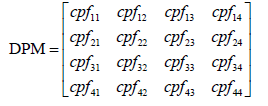In the DPM, totality of all the values in a column is unity.

The interconnected power system error is termed as Area Control Error (ACE). This occurs due to the deviation between scheduled and actual power generation. The ACE of any interconnected power system is given by:

ACE=Δtie, i+biΔfi (1)

Where Δtie, i is the tie-line power change, bi is the frequency bias constant and Δfi is the frequency deviation.

In the deregulated power system, when the load on a particular area changes, error is generated . Since two GENCOs are there in the area considered, in addition to the contribution, the ACE signal should be shared by them. The sharing of ACE by all the four GENCOs are denoted by coefficients called ACE participation factors (apfs). The apf_matrix is denoted as: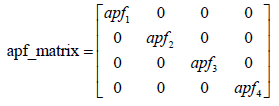apf elements in an area can add up to unity.

The power contracted by DISCOs in area 1 is ΔL1-cont and ΔL2-cont. In area 2 it is represented as ΔL3-cont and ΔL4-cont.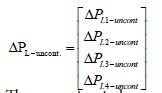The uncontracted power by various DISCOs is denoted as: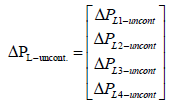Therefore, total power demand in a deregulated power system is:

ΔLL-contL-uncont (2)

Contracted powers from GENCOs in area 1 and 2 are indicated by the ΔG-cont matrix.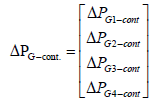The power generated in the two area deregulated power system as per the contract can be calculated using the following equation.

ΔPG-cont=DPM ΔPL-cont (3)

Similarly uncontracted generated power can be calculated from the uncontracted demand and apf-matrix.

ΔG-uncont=apf_matrix ΔPL-uncont (4)

Therefore, total power in the two area deregulated power system to make the frequency error zero is the additive of contracted and uncontracted generated power and is represented as:

ΔGG-contG-uncont (5)

The scheduled steady state power flow in the two area power system on the tie-lines in area i, area j is represented by the following equation.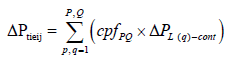(6)

where p represent the pth GENCO of area Ai,q represent the qth DISCO of area Aj,P and Q represent the number of GENCOs and DISCOs of area Ai and Aj respectively. P=2 and Q=2 in the proposed system.

The tie-line power flows scheduled between areas 1 and 2 aregiven by the following relations.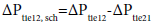(7)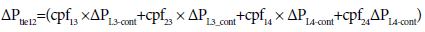(8)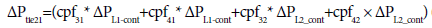(9)

The schematic representation of the proposed two area deregulated power system is given in Figure 2.

#### Controller Design

PI controller

The design of the Proportional Integral controller was aimed at reducing the frequency oscillations of the deregulated two area power systems. In the model of the power system considered using PI controller, the proportional integral control scheme was used to test the performance. The inputs to the controller are ACEi and ∫ACEi and the output of the controller is ui and is given as:

ui=-KpACEi- ∫Ki(ACEi) dt

=-Kptie,i + biΔfi) - ∫Kitie, i+bfi)) dt (10)

Where Kp, Kirepresent the PI controller gains . The Ziegler Nichol’s method is used to tune the gains of the PI controller.

PSO optimized FLC scheme

The FLC is a useful control procedure used in application of nonlinear and indefinite models . Fuzzy logic is very close to natural language and human intuition than traditional frameworks. In the case of complex non-linear systems conventional methods are not suitable and they do not give proper solutions. Conventional controllers perform well only on linear models. Since the proposed system is highly non-linear FLC is considered.

The processes involved in FLC are fuzzification, fuzzy inference and defuzzification . In the fuzzy inference step, fuzzy if-then rules are created from experience. The output from fuzzy inference step is converted into a real value in the defuzzification step. In the proposed work a Multiple-Input-Single-Output (MISO) model FLC is designed and it comprises two inputs ACEi and integral of ACEi and one control output.

Scaling factor tuning in a fuzzy controller is a time-consuming process. This paper proposes an implementation of the PSO technique to tune the scaling factors in a fuzzy controller. For the system considered, the inference mechanism and the membership functions (MFs) have seven levels . There are seven linguistic variables considered for each input in order to achieve better performance. Gaussian membership function is used to identify each variable. Since each variable has seven levels a total of 49 rules are derived. The rule base is formulated in accordance with mamdani type fuzzy IF-THEN rules. The appropriate rules for the inputs ACE and ∫ACE are shown in Figure 3. The MFs of the controller are depicted in Figure 4.

In the figure, the MFs are named Negative Big (NB), Negative Medium (NM), Negative Small (NS), Zero (ZE), Positive Small (PS), Positive Medium (PM) and Positive Big (PB).

The MFs of a Mamdani-type fuzzy controller are tuned by optimally varying the scaling factors of FLC. PSO algorithms are evolutionary algorithms which are successfully applied to many non-linear optimization problems for achieving higher accuracy in lesser time. In the proposed algorithm, the seven linguistic variables of Gaussian type are taken for both inputs ACE and ∫ACE and for the control output variable.

The objective function for total error over the specified time period is taken as:

F=∫ACEi × t dt (11)

In PSO the values associated with the particles are treated as a possible optimal solution to the problem considered . Here a group of random set of populations is chosen for the particles. In every iteration, each particle in the swarm moves towards the personal best arrangement represented as “pbest”. The global best value is denoted by “gbest”.

The PSO velocity change of each particle is represented by the following equation.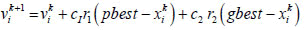(12)

Similarly the position change is represented by the equation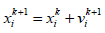(13)

Where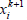=modified position of particle i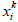=Current position of particle i at iteration k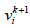=modified velocity of particle i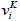=Current velocity of particle i at iteration k

gbest=Global best,

pbest=Personal best of particle i

c1=Positive constant denoting cognitive learning rate

c2=Positive constant denoting social learning rate

Taking inertia weight into account the velocity equation is modified as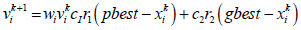(14)

The updated weight is given by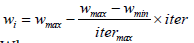(15)

Where

iter.itermax are the current and maximum iteration numbers

wi=Weight function for velocity of particle i

The position and velocity are updated for all iterations as in equations (13) and (14).However it remains unchanged for each particle if its value at the present iteration is better than the previous iteration . This concept of position and velocity update improves computational efficiency.

Proposed algorithm

The PSO algorithm used for tuning the FLC is shown by means of a flow chart in Figure 5.

#### Simulation Studies and Performance Evaluation

Analysis of the deregulated two areas thermal power system is carried out by taking into account two generating and distribution companies in the area considered. The model is generated using simulink and fuzzy logic toolbox of MATLAB. The proposed model is analysed for step load disturbances by PI controller, non-tuned FLC and PSO tuned FLC. The output is limited as 0.15 p.u. The system parameters and their values  are given in appendix I. Three different cases by varying the apf_matrix and distribution company participation matrices are discussed as given below.

Case 1

Consider the case where DISCOs in area 1 alone is demanding power from their local GENCOs. Equal participation of GENCOs take place in AGC and their apf_matrix components are apf1=0.5, apf2=0.5, apf3=0.5 and apf4=0.5. In this case load variation in area 1 is considered. The demanded loads by DISCO1 and DISCO2 are taken as 0.1 p.u. MW. Thus, apf_matrix and DPM for this case is given by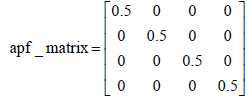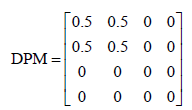Note that in DPM columns 3 and 4 are zero because DISCO3 and DISCO4 do not have any contract with GENCOs. The respective contract participation factors (cpfs) are zero. Here DISCO1 and DISCO2 demand equally from GENCO1 and GENCO2. Figure 6 shows the tie line power and the corresponding frequency deviation. Since the load variation occurs only in area 1, it experiences greater transient frequency deviation than that of area 2. Here, since there is no power contract between GENCO and DISCO in different areas, over the tie line planned steady state power flow is zero.

In any power system under steady state condition, generation and demand should be equal, so that the generation made by GENCO must tally with the demand required by the associated DISCOs. The amount of power generated by a generating company can be represented as the function of total demand of DISCOs and cpfs as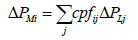(16)

Where ΔLj is the total demand of DISCOj and cpfs are taken as specified by DPM. In the two area deregulated LFC system it is denoted as,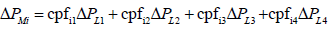(17)

Case 2

In this case the power contract between GENCOs and DISCOs are taken into account. GENCOs in each area take part in AGC and their apf_matrix components are apf1=0.75, apf2=1-apf1=0.25, apf3=0.5 and apf4=1-apf3=0.5. Load variation in area 1 and 2 is also considered Figure 5. The load demanded by all the DISCOs is taken as 0.01 pu MW each. Thus apf_matrix and DPM for this case is given by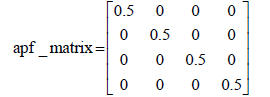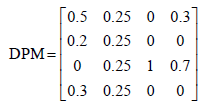The tie line power is represented as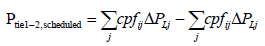(18)

DISCO1 and DISCO2 demand equally from GENCO1 and GENCO2. Figure 7 shows the frequency deviation corresponding to the tie line power, for a step load change in area 1 and 2. In any power system under steady state condition generation and demand should be equal, so that the generation made by GENCO must be balanced by the demand of all associated DISCOs. The amount of power to be generated by a generating company can be represented as the function of the total demand of DISCOs and cpfs as given in eqn (33).

Case 3

In this case, DISCO demands greater power when compared to the contract agreement. This leads to the case of contract violation. The additional power requirement is provided by the GENCOs in the same location. Take all the DISCOs demand as 0.1 p.u MW Additional power requirement of DISCO1 is 0.1 pu MW.

At steady state, the frequency error settles to zero. As the DPM in case 2 and 3 are the same and the excess power requirement of DISCO1 is compensated by GENCO1 located in area 1. Case 2 has the same tie line power. The excess power demand of DISCO1 is shown only on power generation made by GENCO1 and GENCO2. Hence it is clear that in steady state the distribution of additional load of DISCO is determined by the ACE participation factors. The results are shown in Figure 8.

#### Conclusion

To demonstrate the proposed system a deregulated power system with two areas is taken into account. A PSO tuned fizzy logic controller is formulated to improve the overall performance. It is compared with traditional PI controller and non-tuned FLC. The PSO technique can be easily incorporated in the tuning of the controller without difficulty. The controller performance is evaluated by considering settling time, peak undershoot and peak overshoot. Three different cases were carried out and the output shows that the PSO-tuned controller delivers commendable execution when contrasted with the PI and non-tuned FLC. The effectiveness of the proposed system was tested for multiple operating conditions and was found to be superior.

#### References

Select your language of interest to view the total content in your interested language

### Article Usage

• Total views: 2964
• [From(publication date):
December-2016 - Dec 07, 2019]
• Breakdown by view type
• HTML page views : 2730Can't read the image? click here to refresh